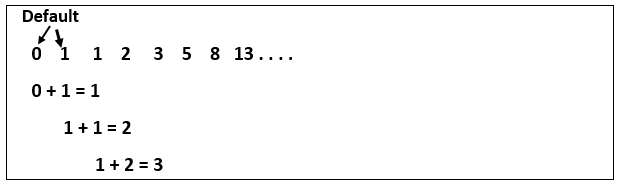Q:

# Python Program to Print the Fibonacci sequence

In this tutorial, we will discuss how the user can print the Fibonacci sequence of numbers in Python.

Fibonacci sequence:

Fibonacci sequence specifies a series of numbers where the next number is found by adding up the two numbers just before it.0, 1, 1, 2, 3, 5, 8, 13, 21, 34, 55, 89, 144, … and so on.

In mathematical terms, the sequence "Fn" of the Fibonacci sequence of numbers is defined by the recurrence relation:

Fn= Fn_1+ Fn_2

Where seed values are:

F0=0 and F1=1

### Method: 1 - By using a while loop

We will use a while loop for printing the sequence of the Fibonacci sequence.

Step 1: Input the number of values we want to generate the Fibonacci sequence

Step 2: Initialize the count = 0, n_1 = 0 and n_2 = 1.

Step 3: If the n_terms <= 0

Step 4: print "error" as it is not a valid number for series

Step 5: if n_terms = 1, it will print n_1 value.

Step 6: while count < n_terms

Step 7: print (n_1)

Step 8: nth = n_1 + n_2

Step9: we will update the variable, n_1 = n_2, n_2 = nth and so on, up to the required term.

``````n_terms = int(input ("How many terms the user wants to print? "))

# First two terms
n_1 = 0
n_2 = 1
count = 0

# Now, we will check if the number of terms is valid or not
if n_terms <= 0:
print ("Please enter a positive integer, the given number is not valid")
# if there is only one term, it will return n_1
elif n_terms == 1:
print ("The Fibonacci sequence of the numbers up to", n_terms, ": ")
print(n_1)
# Then we will generate Fibonacci sequence of number
else:
print ("The fibonacci sequence of the numbers is:")
while count < n_terms:
print(n_1)
nth = n_1 + n_2
# At last, we will update values
n_1 = n_2
n_2 = nth
count += 1  ``````

Output:

```How many terms the user wants to print?  13
The Fibonacci sequence of the numbers is:
0
1
1
2
3
5
8
13
21
34
55
89
144
```

Explanation:

In the above code, we have stored the terms in n_terms. We have initialized the first term as "0" and the second term as "1".

If the number of terms is more than 2, we will use the while loop for finding the next term in the Fibonacci sequence by adding the previous two terms. We will then update the variable by interchanging them, and it will continue with the process up to the number of terms the user wants to print.

Conclusion

In this tutorial, we have discussed how the user can print the number of fibonacci sequence of numbers to the nth terms.